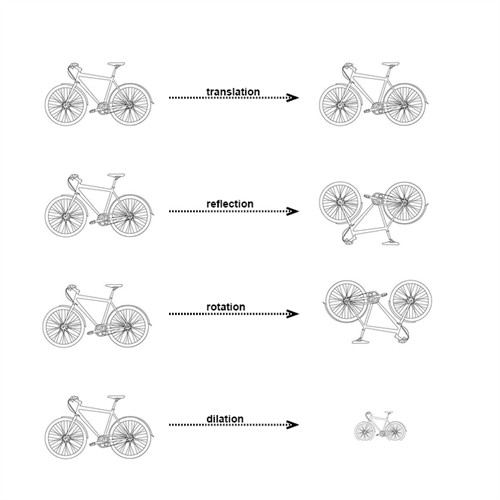# Common types of transformation

Any image in a plane could be altered by using different operations, or transformations. Here are the most common types:Translation is when we slide a figure in any direction.

Reflection is when we flip a figure over a line.

Rotation is when we rotate a figure a certain degree around a point.

Dilation is when we enlarge or reduce a figure.

## Video lesson

Determine which of the triangles that is a reflection, a rotation and a dilation